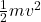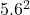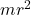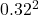## You need to find the moment of inertia of a large wheel about an axis through its center. The radius of the wheel and its mass are 0.320 m a

Question

You need to find the moment of inertia of a large wheel about an axis through its center. The radius of the wheel and its mass are 0.320 m and 30.0 kg, respectively. You design an experiment in which you mount the wheel, using frictionless bearings, on a horizontal axis through the wheel’s center. Then, you wrap a light string around the wheel and hang a 7.00-kg block from the free end of the rope. When you release the mass from rest from 2.50 m above the ground, the wheel turns as the rope unwinds and the block has a speed 5.60 m/s just before it hits the ground.
A) Use energy considerations to find the rotational kinetic energy of the wheel at the moment the block is about to hit the ground.
B) What is the mo of inertia of the wheel you found from this experiment?
C) Is it possible to build a wheel that has a moment of inertia 10 kg.m2 with the mass and the radius given above? Explain.

in progress 0
6 months 2021-08-25T18:11:26+00:00 1 Answers 2 views 0

a) 109.76 J

b) 3.07 kg-m^2

c) no!

it is not possible to build a wheel with moment of inertia of 10 kg-m^2, with the same mass and radius of the wheel.

Explanation:

Radius of the wheel = 0.32 m

mass of the wheel = 30 kg

hanged block mass = 7 kg

height of fall of mass = 2.5 m

final velocity of travel v = 5.6 m/s

initial velocity u = 0 (body is released from rest)

a) According to the energy conservation principle, the kinetic energy of the falling mass will be transformed into the rotational kinetic energy of the wheel

kinetic energy of the falling body ==x 7 x= 109.76 J

b) moment of inertia I of the wheel =m is mass of the wheel,

r is the radius of the wheel

I = 30 x= 3.07 kg-m^2

c) no!

it is not possible to build a wheel with moment of inertia of 10 kg-m^2, with the same mass and radius of the wheel